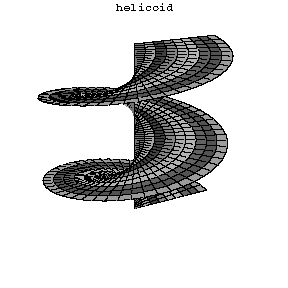# Helicoid

Jump to: navigation, search

2010 Mathematics Subject Classification: Primary: 53A10 Secondary: 49Q05 [MSN][ZBL]

A ruled surface described by a straight line that rotates at a constant angular rate around a fixed axis, intersects the axis at a constant angle $\alpha$, and at the same time becomes gradually displaced at a constant rate $k$ along this axis. The equation of a helicoid in parametric form is $x = \rho \cos t \quad y = \rho \sin t \quad z = \rho \arctan \alpha + kt\, .$ If $\alpha = \pi/2$ the helicoid is called straight or right (cf. Fig. 1), otherwise it is called oblique. However, it is common to use the word "helicoid" without specification for the straight helicoid. Every straight helicoid is a minimal surface, i.e. it has mean curvature zero. In fact the straight helicoid can be characterized by the property of being the only minimal ruled suface. More precisely we have the following

Theorem If $\Sigma\subset \mathbb R^3$ is a connected $2$-dimensional ruled minimal surface, then it is a subset of a straight helicoid or a subset of a plane.

Locally the helicoid is isometric to the catenoid. In fact such local isometry can be achieved as endpoint of a continuous one-parameter family of isometric deformations which are all minimal surfaces.Figure 1: Straight helicoid

How to Cite This Entry:
Helicoid. Encyclopedia of Mathematics. URL: http://encyclopediaofmath.org/index.php?title=Helicoid&oldid=32360
This article was adapted from an original article by A.B. Ivanov (originator), which appeared in Encyclopedia of Mathematics - ISBN 1402006098. See original article